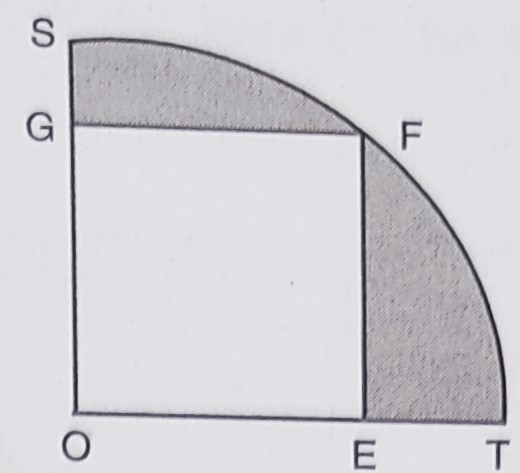"
">

# In the below figure, $O E=20 \mathrm{~cm}$. In sector OSFT, square OEFG is inscribed. Find the area of the shaded region."

Given:

$O E=20 \mathrm{~cm}$.

In sector OSFT, square OEFG is inscribed.

To do:

We have to find the area of the shaded region.

Solution:

Length of the side of the square $s= 20\ cm$

This implies,

Diagonal of the square $=\sqrt{2} \times s$

$=\sqrt{2} \times 20$

$=20 \sqrt{2} \mathrm{~cm}$

Radius of the sector $r=20 \sqrt{2} \mathrm{~cm}$

Therefore,

Area of the quadrant $OTFS =\frac{1}{4} \times \pi r^{2}$

$=\frac{1}{4}(3.14) \times(20 \sqrt{2})^{2}$

$=\frac{1}{4} \times 3.14 \times 800$

$=628 \mathrm{~cm}^{2}$

Area of the square $OEFG=s^2$

$=(20)^{2}$

$=400 \mathrm{~cm}^{2}$

Area of the shaded region $=$ Area of the quadrant $-$ Area of the square

$=628-400$

$=228 \mathrm{~cm}^{2}$

The area of the shaded region is $228\ cm^2$.

Updated on: 10-Oct-2022

32 Views